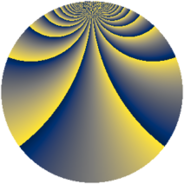# Properties

 Label 1380.2.qLevel $1380$ Weight $2$ Character orbit 1380.q Rep. character $\chi_{1380}(827,\cdot)$ Character field $\Q(\zeta_{4})$ Dimension $560$ Sturm bound $576$

# Related objects

## Defining parameters

 Level: $$N$$ $$=$$ $$1380 = 2^{2} \cdot 3 \cdot 5 \cdot 23$$ Weight: $$k$$ $$=$$ $$2$$ Character orbit: $$[\chi]$$ $$=$$ 1380.q (of order $$4$$ and degree $$2$$) Character conductor: $$\operatorname{cond}(\chi)$$ $$=$$ $$1380$$ Character field: $$\Q(i)$$ Sturm bound: $$576$$

## Dimensions

The following table gives the dimensions of various subspaces of $$M_{2}(1380, [\chi])$$.

Total New Old
Modular forms 592 592 0
Cusp forms 560 560 0
Eisenstein series 32 32 0

## Trace form

 $$560q - 8q^{6} + O(q^{10})$$ $$560q - 8q^{6} - 16q^{12} - 16q^{13} - 8q^{16} - 12q^{18} - 16q^{25} + 24q^{36} - 32q^{46} - 44q^{48} + 64q^{52} + 8q^{58} - 8q^{70} - 36q^{72} - 16q^{73} + 20q^{78} - 96q^{81} - 32q^{82} - 16q^{85} + 64q^{93} - 8q^{96} + O(q^{100})$$

## Decomposition of $$S_{2}^{\mathrm{new}}(1380, [\chi])$$ into newform subspaces

The newforms in this space have not yet been added to the LMFDB.